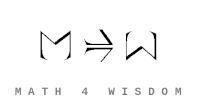文章 发现 ms@ms.lt +370 607 27 665 My work is in the Public Domain for all to share freely. 读物 书 影片 维基百科 Software Upload Understand type theory in terms of amounts and units. Understand type theory Understand logic, proofs, propositions in terms of type theory. When are two types the same? Contraction, exchange, etc. are simply conditions that come from expressing sets as lists. What is gained by a list? Integers. Why integers? Develop my own perspective Develop my ideas on answers, amounts, units in terms of type theory. Develop my ideas on variables and their manifestations in terms of type theory. Understand the equation X=X in terms of ideas of equality from type theory. Describe the mathematics of names (terms, symbols, labels). Readings Videos Oregon Programming Languages Summer School, 2012: Logic, Languages, Compilation, and Verification Robert Harper. Computational Type Theory. 5 lectures. 2018. Robert Harper. Type Theory Foundations. 5 lectures. 2014. Philip Wadler: Propositions as Types A type indicates the range of values that a variable may have. In type theory, implication is understood as a function (by the Howard-Curry correspondence?) This is what I want to say as regards natural transformations - they set up a function that is a validation of a computation, an implication. Type theory: Every answer has an amount and a unit. The unit arises from measurement. Intuitionistic type theory has 3 finite types: type 0 has no terms and means "false" type 1 has 1 canonical term and means "true" type 2 has 2 terms which are 2 choices Type theory defines incomparables and comparables. Like units are combined (internal relations, sets, inner sums). Unlike unites are listed (orders, explicit external relationships, products). Thus this is the distinction between sets and lists. Commutativity and noncommutativity express this in terms of sums. Thus we are ever going back and forth between modes, external and internal, expressed as modes within modes. Type is question, and term is answer. A type is the precondition for a discussion of equality or inequality. Equality Readings Lambda cube Type theory Extensional vs. intensional view of functions. On Lambda Calculus. The seven virtues of simple type theory https://www.researchgate.net/publication/233365263_On_Inversion_Principles Gentzen's Inversion principle https://en.wikipedia.org/wiki/Proof-theoretic_semantics Mathematically Structured Programming Group Programming in Martin-Loef's Type Theory - Bengt Noerdstrom et al Sylvain Salvati. Lambda-calculus and formal language theory. https://ncatlab.org/nlab/show/alpha-equivalence Two syntactic expressions (types, terms, propositions, contexts, whatever) are equivalent for all purposes if their only difference is the renaming of bound variables. idris2 - programming language for type driven development
Šis puslapis paskutinį kartą keistas September 04, 2021, at 02:49 PM